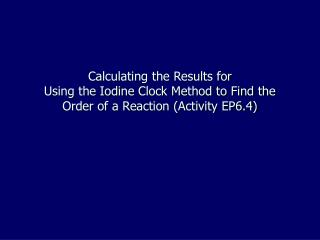Download PresentationCalculating the Results for Using the Iodine Clock Method to Find the Order of a Reaction (Activity EP6.4)# Calculating the Results for Using the Iodine Clock Method to Find the Order of a Reaction (Activity EP6.4) - PowerPoint PPT Presentation

Download Presentation##### Calculating the Results for Using the Iodine Clock Method to Find the Order of a Reaction (Activity EP6.4)

Download Policy: Content on the Website is provided to you AS IS for your information and personal use and may not be sold / licensed / shared on other websites without getting consent from its author. While downloading, if for some reason you are not able to download a presentation, the publisher may have deleted the file from their server.

- - - - - - - - - - - - - - - - - - - - - - - - - - - E N D - - - - - - - - - - - - - - - - - - - - - - - - - - -
##### Presentation Transcript

1. Calculating the Results for Using the Iodine Clock Method to Find the Order of a Reaction (Activity EP6.4)

2. The following series of steps is required to complete the calculations Calculating the concentration of [I-] in the 5 reaction mixtures: Mixture 1: [I-] = Volume of KI x [KI] Total volume of mixture = 5.0 x 0.1 10 = 0.5 mol dm-3 For the other mixtures substitute the appropriate volumes into the above equation

3. Reactant in excess (part c) • The number of mol of I- in mixture 5 is calculated as follows: Concentration = volume of KI x [KI] = 1 x 1.0 = 0.1 mol dm-3 Total volume of mixture 10 • The [S2O82-] in mixture 5 is calculated as follows: Concentration = volume of K2S2O8 x [K2S2O8] = 2 x 0.04 Total volume of mixture 10 = 8 x 10-3 mol dm-3 The calculation shows that I- is in excess. Why is mixture 5 used? It is used because it contains the lowest [KI] used and this can be easily compared to [K2S2O8] which is kept constant in all reaction mixtures

4. Total amount of iodine produced (part d) • This is calculated from the reaction between peroxodisulphate(VI) ions and iodide ions. The reaction for this is: S2O82- (aq) + 2I- (aq) 2SO42- (aq) + I2 (aq) The total moles of iodine produced is calculated as: [K2S2O8]xTotal volume of mixture 1000 = 8 x 10-3 x 10 1000 = 8 x 10-5 moles I2 can be produced

5. The amount in moles of thiosulphate ions added to each mixture (part e i) • This is calculated as : [Na2S2O3]xvolume added 1000 = 0.01 X 2 1000 = 2 x 10-5 moles

6. The amount of iodine used up by thiosulphate ions (part e ii) • The reaction is: 2S2O32- (aq) + I2 (aq) S4O62- (aq) + 2I- (aq) In the reaction 2 moles thiosulphate ions react with 1 mole of iodine. The reaction is 2:1 and the moles of iodine used up is calculated to be: 1 x 10-5 moles

7. The percentage of the reaction studied (part e iii) This is calculated as follows: % Reaction studied = Moles of iodine used x 100 Moles of iodine made = 1 x 10-5 x 100 8 x 10-5 = 12.5%

8. Calculating the initial rate • The can be calculated for each mixture as follows: • Rate = The number of moles of iodine used Time taken for colour to appear (T) Or 1 x 10-5 T

9. Tabulating the results